← Back to Community

Profile icon
RtoonTV

# Introduction.css-1q3m8ps{margin-left:var(--space-4);margin-right:var(--space-4);display:none;}.css-1gcl232{min-width:16px;min-height:16px;}

We coders use different programming languages here and there to command the computer to do certain type of work. Hmm... a question might be arising in your mind that does a computer really understand a programming language? Well... No, Wait a minute before you shout on me that we use programming languages and the computer is doing the work commanded then how it is not understanding it. Well when we write the code and run it the computer can't directly understand the code written rather it converts the code to the language it understands, which is the Binary number system. Confused? Well don't be I am gonna explain you everything in detail; all about the binary number system and other number systems and how to convert from one number system to another and how to perform mathematical operations on binary numbers.

(Disclaimer: In this tutorial I will not be teaching any programming language rather a general concept of Binary numbers.
No coding required)

# Binary Number System

The Binary number system consists of only two digits zero and one (0 and 1). It has the base 2, i.e., it has 2 digits .A base is the number of digits in a number system.

# Why Binary?

A computer cannot understand human language , rather it understands only the binary code. Therefore, the data that is entered into a computer is converted into its binary equivalent. It further converts the binary results into their decimal equivalents for output.

# Conversion Of Decimal Into Binary Number System

The equivalence between binary and decimal numbers can be understood with the given examples. Follow the steps given below-

• Step 1: Divide the given decimal number with the base 2.
• Step 2: Write down the remainder, divide the quotient again by 2.
• Step 3: Repeat step 2 till the quotient is zero.
• Step 4: Remainders, which are obtained in each step are written in reverse order when writing the number.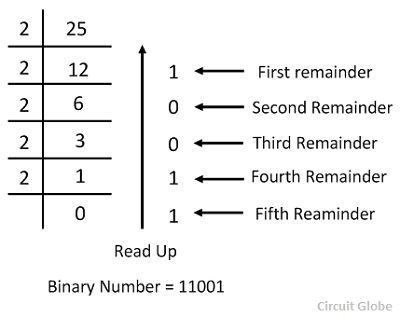### Practice Questions-

Do these practice questions in your book/paper and write and submit your answers in the comments section.

• base 10 means it is a decimal number(by saying decimal number I don't mean that it has the decimal point rather the normal integers (55,-55,0,5.5,etc).The numbers in the bracket are the numbers and numbers outside is the base. Just ignore the numbers outside.
• (25)10 to binary
• (13)10 to binary

# Binary To Decimal

To convert a binary number to decimal, follow the steps given below.

• Multiply each binary number with its positional value, which is in terms of powers of 2,starting from the extreme right digit.
• Increase the power one by one, keeping the base fixed as 2.
• Sum up all products to get the decimal number.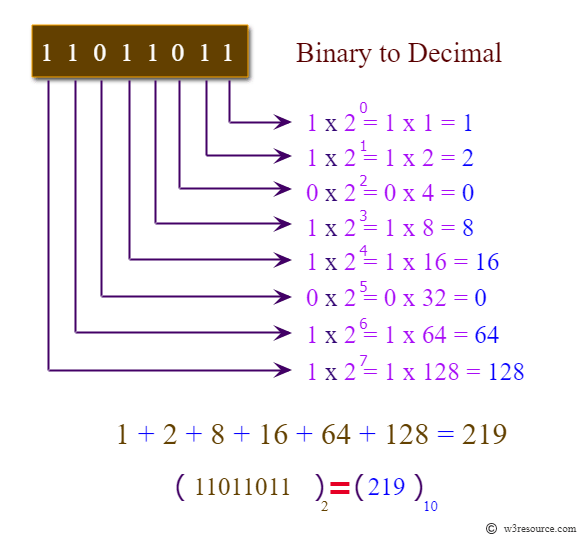### Practice Questions

• (1010)2 to decimal
• (1100)2 to decimal

# Octal Number System

The Octal number system consists of 8 digits: 0 to 7 with the base 8.The procedure of octal to decimal is same as binary to decimal with the difference of base. Instead of base 2 base 8 is used. We wont learn octal to binary since there is no calculation involved in it.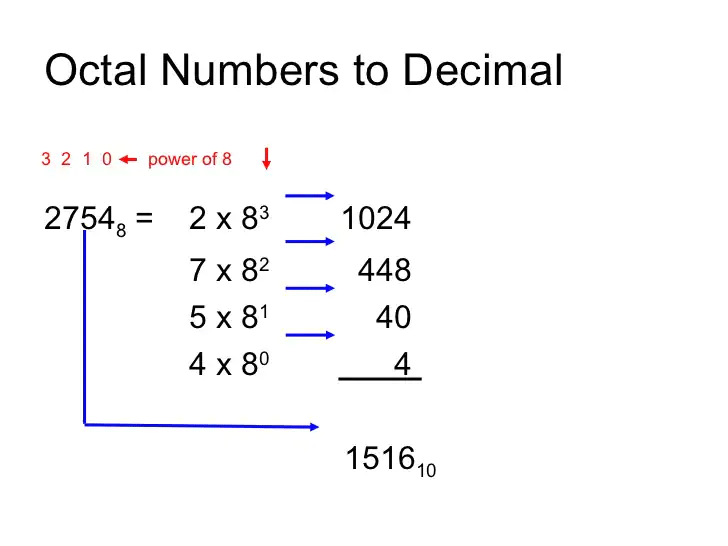### Practice Questions

• (345)8 to decimal
• (317)8 to decimal

# Hexadecimal Number System

This number system consists of 16 digits:0-9 and the letters A-F, where A-F represents digits 10 to 15. This number system has a base 16. Converting hexadecimal to decimal is same as binary to decimal or octal to decimal with the change of base.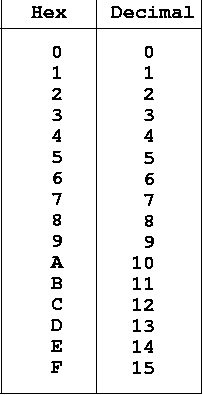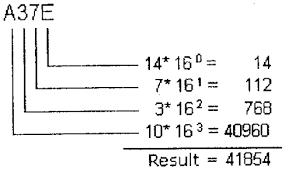Converting hexadecimal to binary is also really simple. Write down the digits seperatly and find their binary equivalent by doing decimal to binary conversion method then join the binary numbers.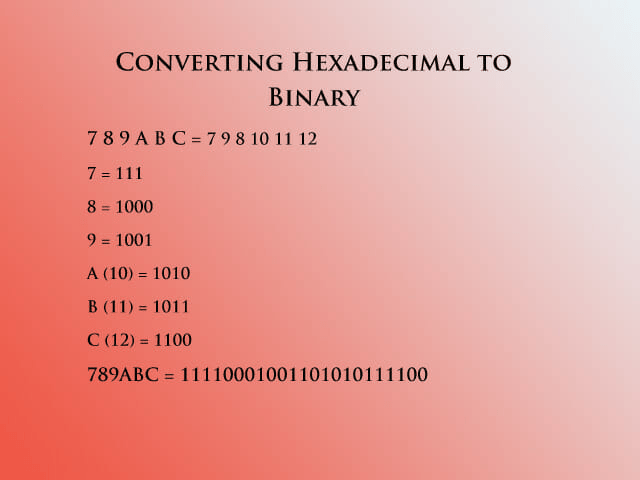### Practice Questions

• (3B3)16 to decimal
• (4D2F7)16 to binary

The technique used to add binary numbers is very easy and simple. This is performed in the same way as you perform addition with decimal numbers.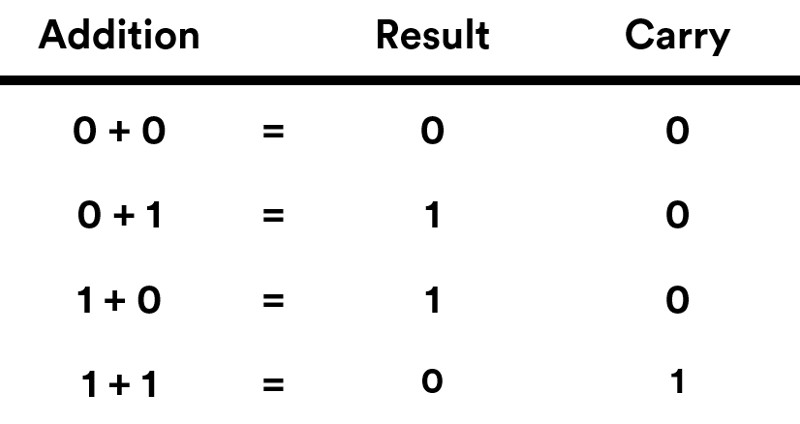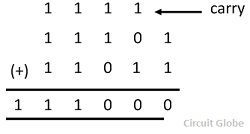### Practice Questions

• add (1000)2 with (111)2
• add (11111)2 with (1011)2

# Binary Subtraction

• Binary subtraction is same as decimal subtraction.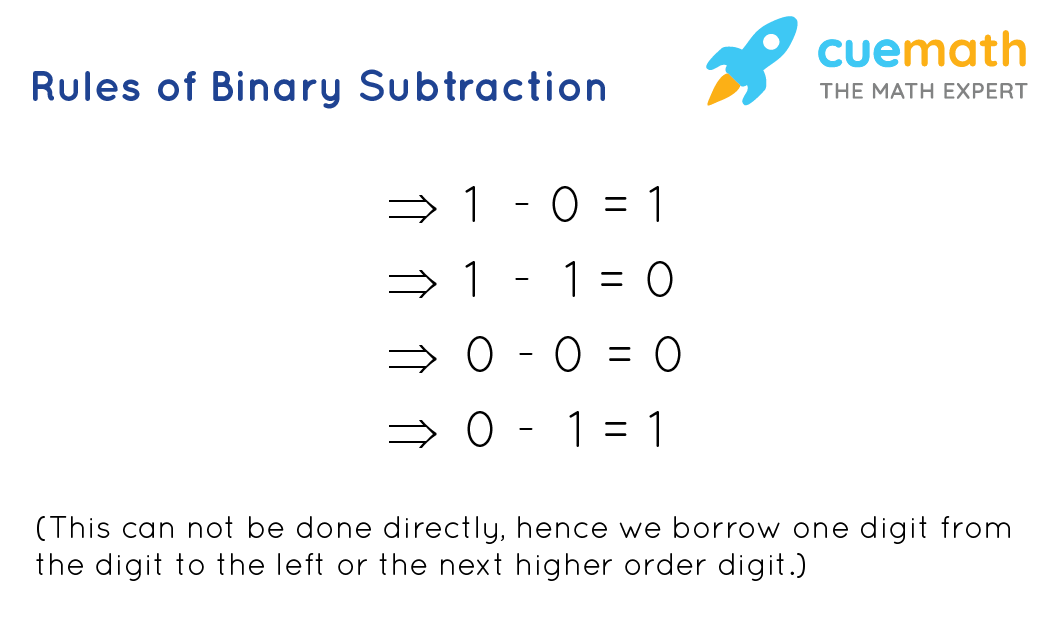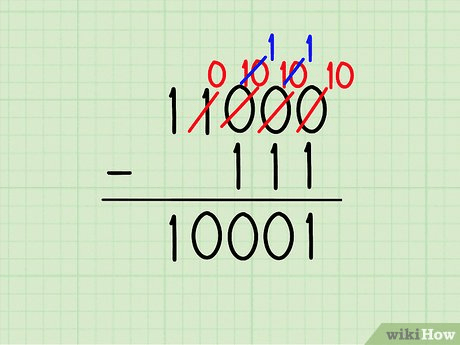### Practice Questions

• subtract (1010)2 from (1111)2
• subtract (11)2 from (1100)2

# Binary Multiplication

The rules for performing binary multiplication is same as decimal multiplication.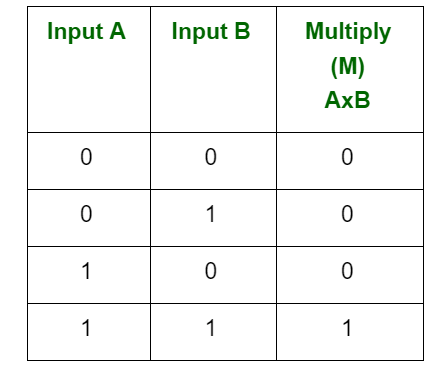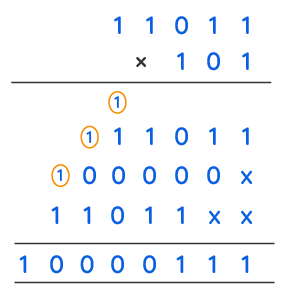### Practice Questions

• (101)2 multiplied by (11)2

# Binary Division

The rules of dividing binary numbers is same as dividing decimal numbers.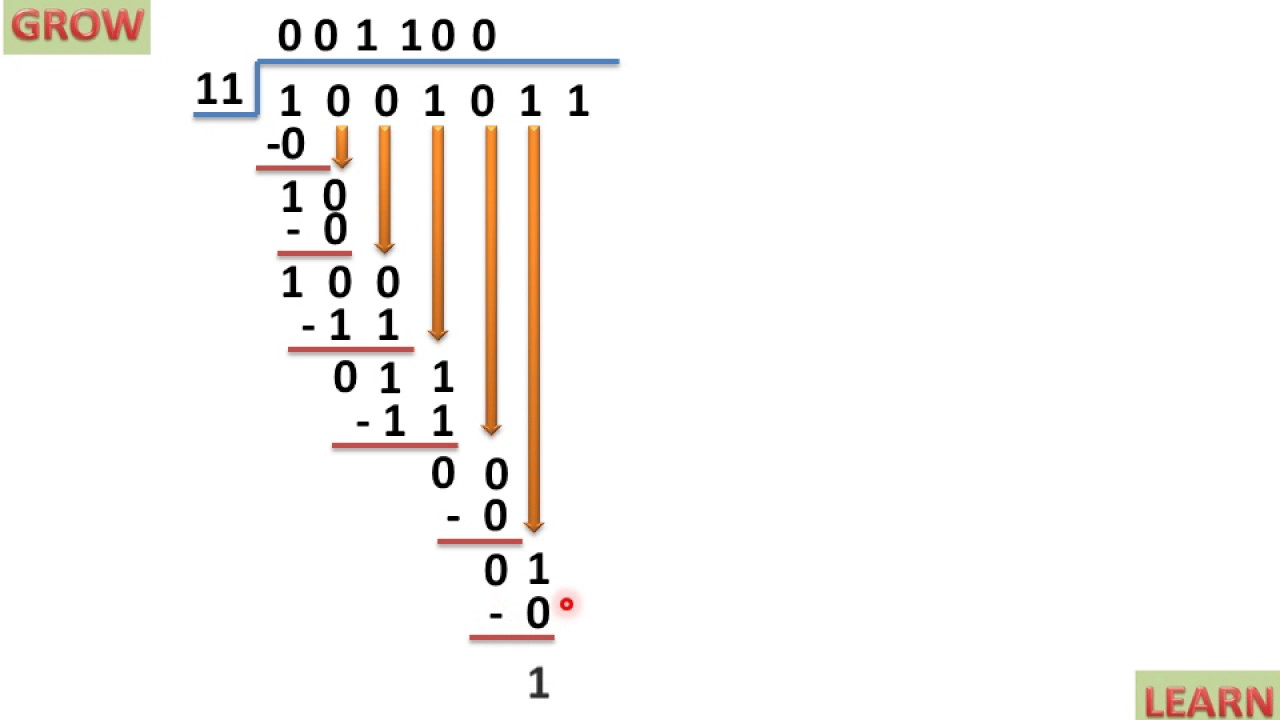### Practice Questions-

• Divide (10011)2 by (101)2

Here we finish our lesson. I hope you all learned something new and enjoyed it. Pls upvote my lesson. If you have any doubt write it in the comments section.

Voters
Profile icon
GrantJohnson
Profile icon
365DaysOfCode
Profile icon
RtoonTV